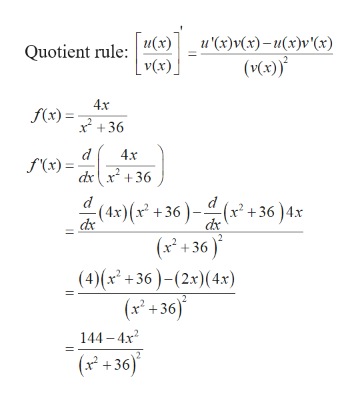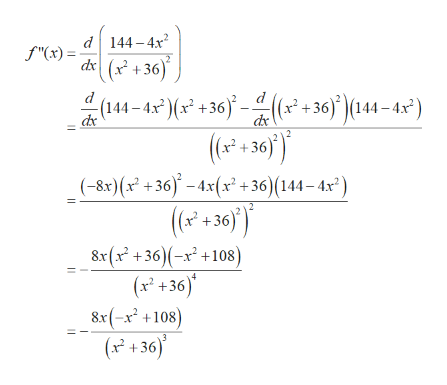# determin where the function is concave up and where ti is concave down. f(x)=4x/(x^2+36)

Question
12 views

determin where the function is concave up and where ti is concave down.

f(x)=4x/(x^2+36)

check_circle

Step 1

Test for Concavity:

If  f "(x) > 0  for all values of x in the interval, the graph is concave upward.

If  f "(x) < 0  for all values of x in the interval, the graph is concave downward.

Step 2

Differentiate the given function.

Here we have to use the Quotient rule.help_outlineImage Transcriptioncloseи(х) u'(x)v(x)-u(x)v°(x) Quotient rule (Vx)* _ v(x) 4x f(x) 36 d f'(x)= 4x dxx+36 ( 4x)( +36)-( +36) 4x (x+36) (4)(x2+36)(2x)(4x) (x+36) 144 4x (x +36) fullscreen
Step 3

Differentiate again to get...help_outlineImage Transcriptionclosed144-4x2 dx(36 f"(x) (144-4x*( +36j <(x+ 36) )(144-4x*) (a2+30') (-8x) (x? +36 =4x(x? + 36)(14- 4x*") (+36) 8x (x2 +36)(-x +108) (x +36) 8x(-x2 +108) (x +36) dк fullscreen

### Want to see the full answer?

See Solution

#### Want to see this answer and more?

Solutions are written by subject experts who are available 24/7. Questions are typically answered within 1 hour.*

See Solution
*Response times may vary by subject and question.
Tagged in

### Calculus